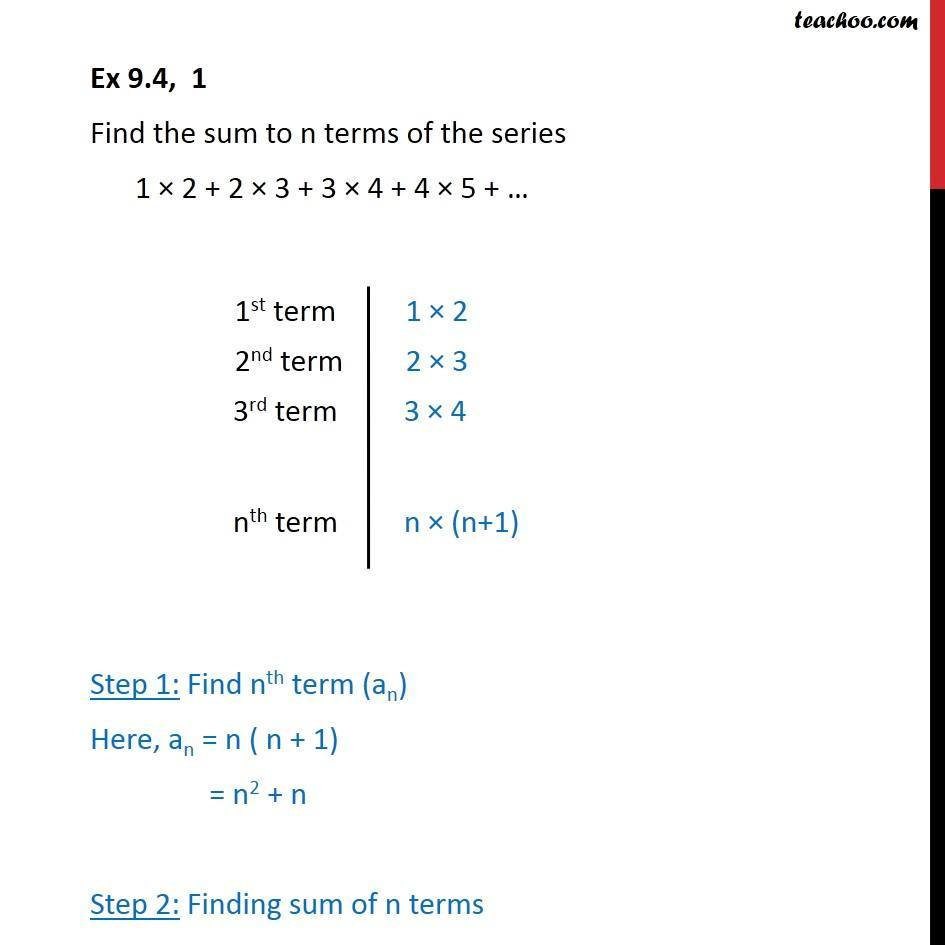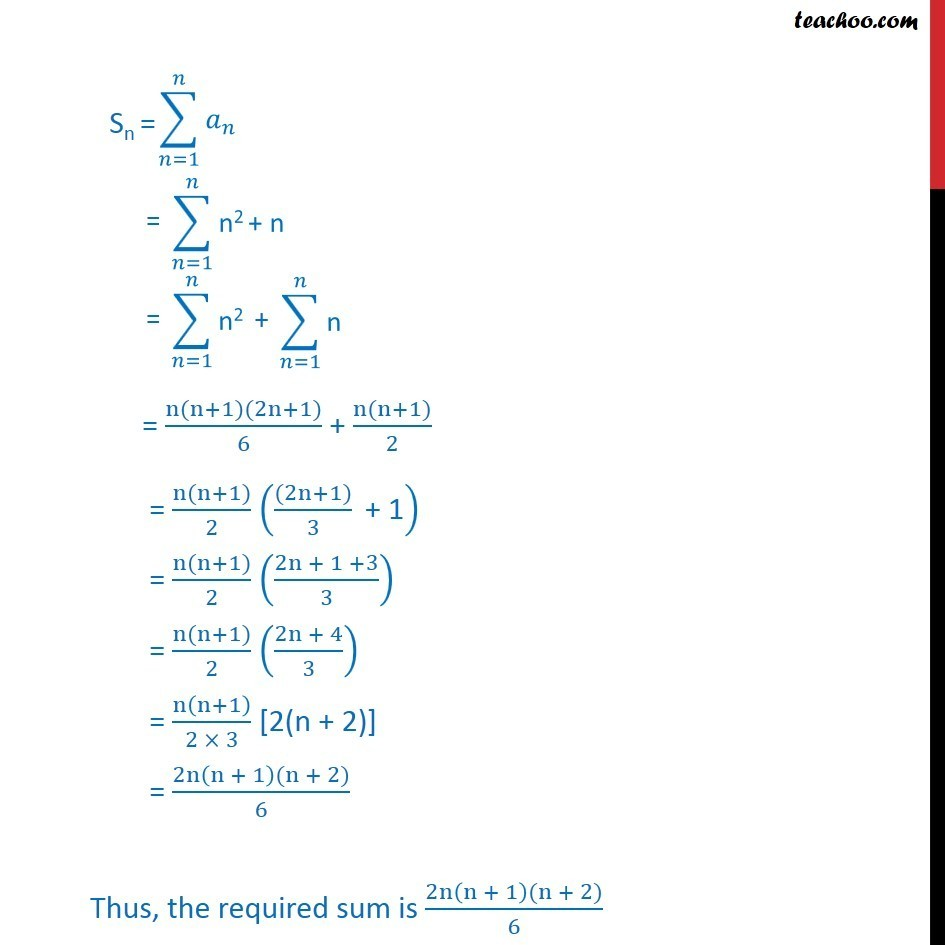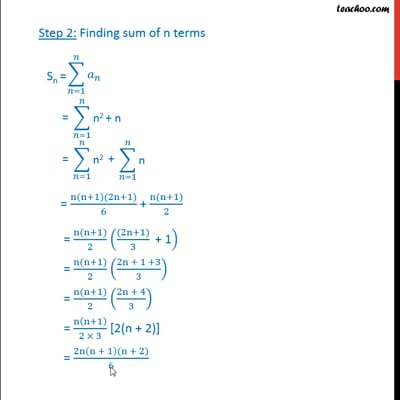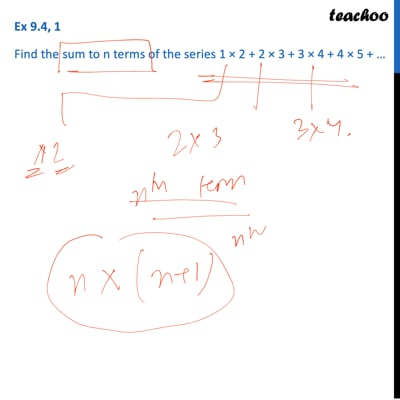Finding sum from nth number

Chapter 8 Class 11 Sequences and Series
Concept wiseThis video is only available for Teachoo black usersThis video is only available for Teachoo black users

Learn in your speed, with individual attention - Teachoo Maths 1-on-1 Class

### Transcript

Question 1 Find the sum to n terms of the series 1 2 + 2 3 + 3 4 + 4 5 + Step 1: Find nth term (an) Here, an = n ( n + 1) = n2 + n Step 2: Finding sum of n terms = (n(n+1)(2n+1))/6 + (n(n+1))/2 = (n(n+1))/2 (((2n+1))/3 " + 1" ) = (n(n+1))/2 ((2n + 1 +3)/3) = (n(n+1))/2 ((2n + 4)/3) = n(n+1)/(2 3) [2(n + 2)] = (2n(n + 1)(n + 2))/6 Thus, the required sum is (2n(n + 1)(n + 2))/6